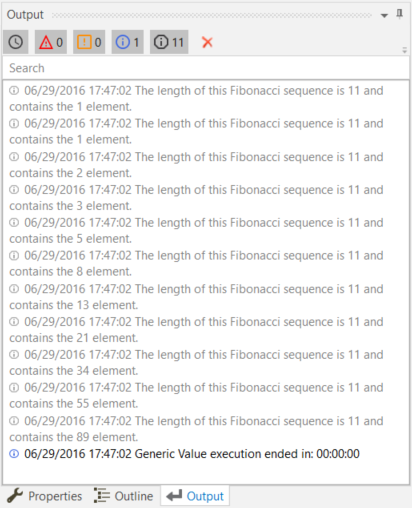Subscribe

# The For Each Activity

The For Each activity enables you to step through arrays, lists, data tables or other types of collections, so that you can iterate through the data and process each piece of information individually.

# Example of Using a For Each Activity

To exemplify how to use a For Each activity, let’s create an automation that goes through each element of an array of integers and writes the length of the array and each element to the Output panel.

1. Create a new sequence.
2. Create an array of integer variables, `arrFibonacciNumbers`.
3. In the Default field, type the Fibonacci sequence up to a desired value, such as {1, 1, 2, 3, 5, 8, 13, 21, 34, 55, 89}.
4. Add a For Each activity in the Designer panel.
5. Do not make any changes to the Foreach field.
6. In the In field, add the `arrFibonacciNumbers` variable. This activity looks at each individual item in the provided variable.
7. In the Body section of the For Each activity, add a Write Line activity.
8. In the Text field, type "The length of this Fibonacci sequence is " + `arrFibonacciNumbers.Length.ToString` + " and contains the " + `item.ToString` + " element.". This expression enables you to write the total number of array elements and each element of the array in the Output panel.

The final project should look as in the following screenshot.1. Press F5. The automation is executed. Note that the Output panel displays the correct message for each element of the array.

### 📘Note:

The Length property enables you to find out the total number of array elements.Updated 3 years ago